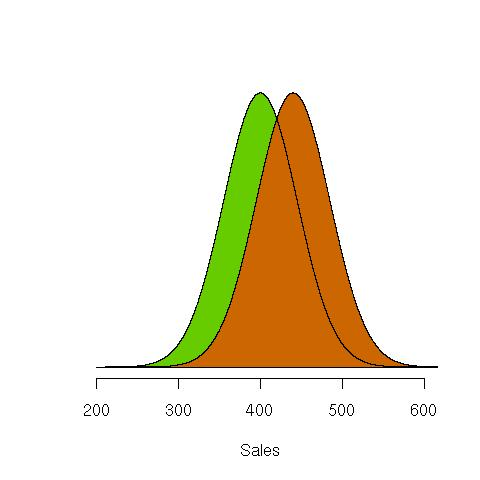# Why most statistics don’t mean what you think they do: Part II.

In Part I of this post, we started with a typical problem: which of two advertising campaigns was “better” in terms of generating more sales. Campaigns A and B were each tested for 20 days, during which time sales data was collected. The mean sales during Campaign A was \$421 and the mean sales during Campaign B was \$440.

Campaign B looks better on this evidence, doesn’t it? But suppose instead of 20 days, we only ran the campaigns one day each, and that the sales for A was just \$421 and that for B was \$440. B is still better, but our intuition tells us that the evidence isn’t as strong because the difference might be due to something other than differences in the ad campaigns themselves. One day’s worth of data just isn’t enough to convince us that B is truly better. But is 20 days enough?

Maybe. How can we tell? This is the part that Statistics plays. And it turns out that this is no easy problem. But please stay with me, because failing to understand how to properly answer this question leads to the most common mistake made in statistics. If you routinely use statistical models to make decisions like this—“Which campaign should I go with?”, “Which drug is better?”, “Which product do customers really prefer?”—you’re probably making this mistake too.

In Part I, we started by assuming that the (observable) sales data could be described by probability models. A probability model gives the chance that the data can take any value. For example, we could calculate the probability that the sales in Campaign A was greater than \$500. We usually write this using math symbols like this:

Pr(Sales in Campaign A > \$500 | e)

Most of that formula should make sense to you, except for the right-hand side of it. The bar at the end, the “|”, is the “given” bar. It means that whatever appears to the right of it is accepted as true. The “e” is whatever evidence we might have, or think is true. We can ignore that part for the moment, because what we really want to know is

Pr(Sales in B > Sales in A | data collected)

But that turns out to be a question that is impossible to answer using classical statistics!

Before I tell what kind of questions classical statistics can answer, we first have to do some fairly hard work. Remember (from Part I) that probability distributions are mathematical formulae that require something called parameters to fully describe them. A parameter is an unobservable mathematical crutch that allows us to index a probability distribution (parameters do not exist in reality). The probability distribution used in these types of problems is the normal (the bell-shaped curve), which requires two parameters, ? (or “mu”) and ?^2 (or “sigma-squared”, which we’ll ignore for this article).

The first, ?, is (or should be) called the central parameter: it gives us the point of the data that is the most likely. Obviously, then, higher values of ? correspond to greater likelihoods of larger data; which here means larger sales. We want a probability distribution for sales data in both campaigns, so we need to know both ?_A and ?_B.

The following picture shows two normal distributions one (in green) has ?=400 and the other (in orange) ?=440. It’s easy to see that the orange curve gives more weight (has a line which is higher) to higher sales than does the green curve.The difficulty is that we do not know the value of ?! If we did, then we could look at the value of ?_A and that of ?_B and then pick the campaign that had the higher value, because that would naturally imply higher sales data for that campaign.

We do know, however, how to make a guess at the values of ?_A and ?_B. But since they are guesses, we cannot be sure that the values we pick are correct. So we have to have some measure of our certainty that we have chosen the right numbers. Or, even more importantly, some measure of certainty that the decision we make is the correct one.

It turns out that there are three ways to do this: one terrible, and the one way which you probably use all the time, one so-so, and one very good method. We’ll go over them all next time.

Categories: Uncategorized